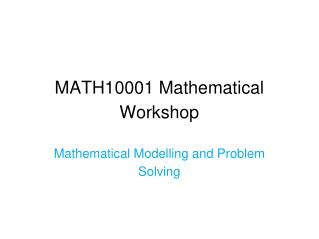Download PresentationMATH10001 Mathematical Workshop

# MATH10001 Mathematical Workshop - PowerPoint PPT Presentation

MATH10001 Mathematical Workshop. Mathematical Modelling and Problem Solving. Traditional view of maths?. Maths is useless The only jobs maths can be used for are accountancy and teaching Maths has no link with the real world. The truth is that mathematicians have changed the world,I am the owner, or an agent authorized to act on behalf of the owner, of the copyrighted work described.
Download Presentation## MATH10001 Mathematical Workshop

Download Policy: Content on the Website is provided to you AS IS for your information and personal use and may not be sold / licensed / shared on other websites without getting consent from its author.While downloading, if for some reason you are not able to download a presentation, the publisher may have deleted the file from their server.

- - - - - - - - - - - - - - - - - - - - - - - - - - E N D - - - - - - - - - - - - - - - - - - - - - - - - - -
Presentation Transcript
1. MATH10001 Mathematical Workshop Mathematical Modelling and Problem Solving

2. Traditional view of maths? • Maths is useless • The only jobs maths can be used for are accountancy and teaching • Maths has no link with the real world

3. The truth is that mathematicians have changed the world, Radio, digital revolution Engineering Computers Security Health

4. TelecommunicationsFourier • Fourier Transforms • Joseph Fourier, 1768-1830 • Mobile phones, digital radios, MP3 players etc. all use these ideas. • , MP3 players etc all use the sameideas

5. Internet shopping • Internet transfer protocols based on mathematics • Credit cards and online shopping • Modern encryptionalgorithms like RSAuse prime number theorems

6. Scanners • Security screening at the airport • MRI scanners in hospitals

7. Mathematical modelling in engineering: eg. Finite element modelling to reduce vibration Divide car into small cuboids/tetrahedra. Treat it a bit like masses and springs in a network. Vibrations modelled using eigenvalues of matrices.

8. Traffic Management • Variable speed signs • “shock waves” • Many models of traffic flow • Road design • Traffic control • Better throughput • Better safety

9. modelling Mathematical Model Real World problem solving Modelling Cycle testing Explanations &Predictions Solution interpretation

10. Steps in the modelling cycle • Identify the problem • Define the variables and parameters • Variables are quantities that can change in a problem • Parameters are quantities that remain constant • Make assumptions • Write down a model - relationships between variables • Solve the model • Test the solution against the real life problem • Refine the model

11. Example – modelling the growth of bacteria We start with 500 bacteria in a Petri dish. After one day we have 525 bacteria, after two days we have 551 bacteria. • The problem is to find a formula for the number of bacteria after n days. • Variables include the population, growth rate, time, temperature, amount of food, amount of space left in dish etc. Parameters include initial population, size of dish, initial amount of food. • To simplify the problem we make certain assumptions – ignore the amount of food, temperature and space in dish and assume that the growth rate is constant.

12. 4. Define the model: first introduce some notation: We write down the relationship We have Assuming a growth rate of 0.05 (from our observations) we get

13. 6. Test the solution: we test our solution against observations. We can see that the model works well at the start but after 6 days the model is not accurately predicting the population. This tells us that we need to modify our model.

14. 7. The growth rate appears to be decreasing over time. This could be due to a change in food available or room to grow. We chose to ignore those variables in the original model. As the growth rate is changing we could replace our constant rate by one which is a function of n. Why do we need mathematical models? Why don’t we simply make lots of observations?